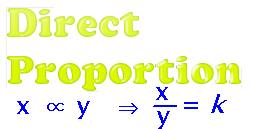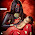## Wednesday, 13 March 2013

### CBSE Class 8 - Maths - CH13 - Direct and Inverse Proportions (Ex 13.1)

Direct and Inverse Proportions

An increase (↑) in one quantity brings about an increase (↑) in the other quantity and similarly a decrease (↓) in one quantity brings about a decrease (↓) in the other quantity, the these two quantities are in direct proportion or direct variation.

In direct proportion the ratio of two quantities (say x and y) is a constant called constant of proportionality.
⇒ y/x = k          or       y = k × x

NCERT Chapter Solutions and other Q & A

NCERT Ex. 13.1

Q1: Following are the car parking charges near a railway station upto
4 hours Rs 60
8 hours Rs 100
12 hours Rs 140
24 hours Rs 180

Check if the parking charges are in direct proportion to the parking time

Answer: Calculating the ratio of parking charging wrt parting time:

No. of Hours (a)           Parking Charges(B)        Ratio (B/A)
46060/4 = 15:1
8100100/8 =25:2
12140140/12 =35:3
24180180/24=15:2

Since the ratio of various parking charging vs parking time is not the same, the parking charges are NOT in direct proportion to the parking time.

Q2: A mixture of paint is prepared by mixing 1 part of red pigments with 8 parts of base.
In the following table, find the parts of base that need to be added.

 Parts of red pigment 1 4 7 12 20 Parts of base 8 ... ... ... ...

Answer: With increase in parts of the red pigments, there will be corresponding increase in parts of the base, since the parts are in direct proportion.

 Parts of red pigment 1 4 7 12 20 Parts of base 8 p1 p2 p3 p4

```    p1              8
⇒  -------  = -----
4            1
⇒ p1  = 8 × 4 = 32

p2              8
⇒  -------  = --------
7            1
⇒ p2  = 8 × 7 = 56
p3           8
⇒  -------  = --------
12            1
⇒ p3  = 8 × 12 = 96
p4          8
⇒  -------  = --------
20           1
⇒ p4  = 8 × 20= 160

```

Q3: In Question 2 above, if 1 part of a red pigment requires 75 mL of base, how much red pigment should we mix with 1800 mL of base?

Answer:  Let the red pigment in 1800mL of base = p mL
red pigment in base are in direct proportion and are in ratio = 1:75
∴ p:1800 = 1:75
∴ p/1800 = 1/75
⇒ p = 1800/75 = 24
Thus 24 parts of red pigment should be mixed with  1800mL of base.

Q4: A machine in a soft drink factory fills 840 bottles in six hours. How many bottles will it fill in five hours?

Answer: A machine in soft drink factory fills 840 bottles in six hours.
Less hours means less number of bottles and more hours means more bottles will be filled in.
∴ Number of hours is in direct proportion with number of bottles.
Let number of bottles to be filled in five hours = n
⇒ n:5 = 840:6
⇒ n/5 = 840/6
⇒ n = 840 × 5/6 = 700
Thus in five hours, 700 bottles will be filled in.

Unitary Method:
In 6 hours, the machine fills in = 840 bottles
In 1 hours, the machine fills in = 840/6
In 5 hours, the machine fills in = 840 × 5/6 = 700

Thus in five hours, 700 bottles will be filled in.

Q5: A photograph of a bacteria enlarged 50,000 times attains a length of 5 cm as shown in the diagram. What is the actual length of the bacteria? If the photograph is enlarged 20,000 times only, what would be its enlarged length?

Answer:   No. of times the photograph is enlarged, more will the length of the photograph.
⇒ Length of photograph is in direct proportion with Enlargement of the photograph.
⇒ Length of photograph(l) ∝ no. of times photo is enlarged.
⇒ 5cm ∝ 50000
or 5:50000 = x:20000
∴ x = 20000 × 5 / 50000 = 2 cm
∴ Photograph when enlarged 20,000 times will have length of 2cm.

Q6: In a model of a ship, the mast is 9 cm high, while the mast of the actual ship is 12 m high. If the length of the ship is 28 m, how long is the model ship?

Answer: The model dimensions are in direction proportion to actual dimensions of the ship.
⇒ length of mast in model:length of mast of ship = length of model ship(x) : length of actual ship
⇒ 9:12 = x:28
⇒ x = 28 × 9 /12 = 21 cm
Thus length of model ship is 21 cm.

Q7: Suppose 2 kg of sugar contains 9 × 106 crystals. How many sugar crystals are there in
(i) 5 kg of sugar?
(ii) 1.2 kg of sugar?

Answer: More the sugar weighs, higher the number of sugar crystals.
⇒ number of sugar crystals ∝ weight of the sugar.
(i)
⇒ 2: 9 × 106 = 5:n
⇒ 2/(9 × 106 ) =  5/n
⇒ n = 9 × 106 × 5 /2 = 22.5 × 106 = 2.25 × 107                                   ... answer

(ii) Similarly,
2: 9 × 106 = 1.2:n
⇒   n = 9 × 106 × 5 /1.2 = 5.4 × 106                                                  ... answer

Q8:Rashmi has a road map with a scale of 1 cm representing 18 km. She drives on a road for 72 km. What would be her distance covered in the map?

Answer: Road map scale is in direct proportion to the actual road length.
Let the map length for 72km long road is x cm.
∴ 1cm:18km = x:72 km
⇒ 1/18 = x/72
⇒ x = 72/18 = 4 cm
Thus the map distance is 4 cm for the equivalent road distance of 72 km.

Q9: A 5 m 60 cm high vertical pole casts a shadow 3 m 20 cm long. Find at the same time
(i) the length of the shadow cast by another pole 10 m 50 cm high
(ii) the height of a pole which casts a shadow 5m long.

Answer: (i) Let the length of shadow cast by another pole = x m
Since Shadow length  ∝ pole length
∴  3.20 : 5.60 = x : 10.50
⇒ 3.20/5.60 = x / 10.50
⇒ x = 10.50 × 3.20 / 5.60  = 6
∴ Length of shadow of pole of length 10.50 m is 6 m

(ii) Let the height of the pole = y m
Since Shadow length  ∝ pole length
∴  3.20 : 5.60 = 5 : y
⇒ y = 5 × 5.60 / 3.20 = 8.75m
∴ Height of the pole is 8 metres and 75 centimetres.

Q10: A loaded truck travels 14 km in 25 minutes. If the speed remains the same, how far
can it travel in 5 hours?

Answer: Let the distance travelled by the truck in 5 hours = d km
5 hours  = 5 × 60 min = 300 min
Distance travelled ∝ Time
∴  14 km :25 min = d : 300 min
⇒ 14/25 = d / 300
⇒ d = 14 × 300/25 = 168 km
Thus the distanced travelled in 5 hrs is 168 km.

Miscellaneous Q & A

Q11: If the cost of 16 pencils is 48, find the cost of 4 pencils.

Answer:  If number of files decreases, cost also decreases. It means number of pencils and their cost are in direct proportion.

∴ 16 : 48 = 4 : x
⇒ 16/48 = 4/x
⇒ x = 48 × 4 / 16 =  12

.1.2.